TUTORIALS
TUTORIALS HOME

GENERAL MATH
NUMBER SETS
ABSOLUTE VALUE & INEQUALITIES
SETS & INTERVALS
FRACTIONS
POLYNOMIALS
LINEAR EQUATIONS
GEOMETRY
FINITE SERIES
TRIGONOMETRY
EXPONENTS
LOGARITHMS
INDUCTION

CALCULUS
LIMITS
DERIVATIVES
RELATED RATES & OPTIMIZATION
CURVE SKETCHING
INTEGRALS
AREA & VOLUME
INVERSE FUNCTIONS

MAIN
HOME
TESTS
TUTORIALS
SAMPLE PROBLEMS
COMMON MISTAKES
STUDY TIPS
GLOSSARY
CALCULUS APPLICATIONS
MATH HUMOUR

A quadratic equation is a trinomial of the form ax2 + bx + c = 0. There are three main ways of solving quadratic equations, that are covered below.

# Factoring

Review the factoring sections of polynomials tutorial

If a quadratic equation can be factored, then it can be written as a product of two binomials. Since the trinomial is equal to 0, one of the two binomial factors must also be equal to zero. By factoring the quadratic equation, we can equate each binomial expression to zero and solve each for x.

# Examples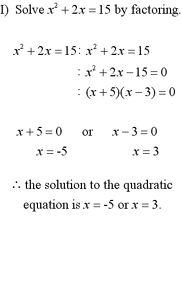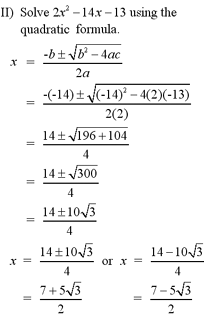# Completing the Square

Quadratic equations cannot always be solved by factoring. They can always be solved by the method of completing the squares. To complete the square means to convert a quadratic to its standard form. We want to convert ax2+bx+c = 0 to a statement of the form a(x - h)2 + k = 0. To do this, we would perform the following steps:

1) Group together the ax2 and bx terms in parentheses and factor out the coefficient a.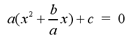2) In the parentheses, add and subtract (b/2a)2, which is half of the x coefficient, squared.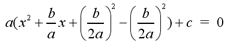3) Remove the term - (b/2a)2 from the parantheses. Don't forget to multiply the term by a, when removing from parentheses.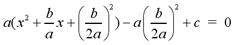4) Factor the trinomial in parentheses to its perfect square form, (x + b/2a)2.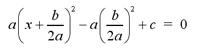Note: If you group the - (b/2a)2 + c terms together in parentheses, the equation will now be in standard form. The equation is now much simpler to graph as you will see in the Graphing section below. To solve the quadratic equation, continue the following steps.

5) Transpose (or shift) all other terms to the other side of the equation and divide each side by the constant a.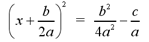6) Take the square root of each side of the equation.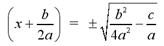7) Transpose the term -b/2a to the other side of the equation, isolating x.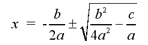The quadratic equation is now solved for x. The method of completing the square seems complicated since we are using variables a,b and c. The examples below show use numerical coefficients and show how easy it can be.

# ExamplesNote: For more examples of solving a quadratic equation by completing the square, see questions #1 and #2 in the Additional Examples section at the bottom of the page.

The method of completing the square can often involve some very complicated calculations involving fractions. To make calculations simpler, a general formula for solving quadratic equations, known as the quadratic formula, was derived. To solve quadratic equations of the form ax2 + bx + c = 0, substitute the coefficients a,b and c into the quadratic formula.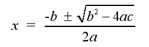The value contained in the square root of the quadratic formula is called the discriminant. If,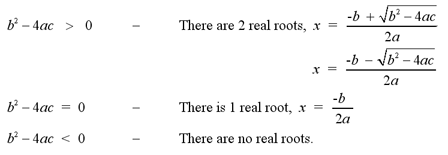# Examples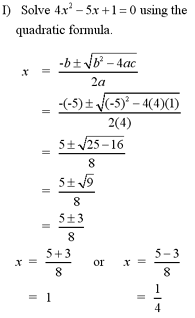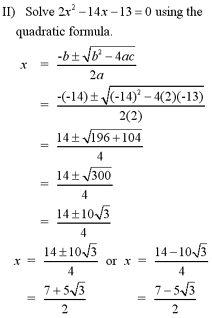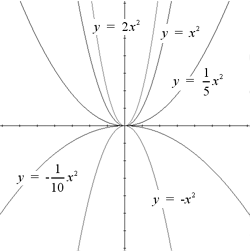The graphical representation of quadratic equations are based on the graph of a parabola. A parabola is an equation of the form y = a x2 + bx + c. The most general parabola, shown at the right, has the equation y = x2.

The coefficent, a, before the x2 term determines the direction and the size of the parabola. For values of a > 0, the parabola opens upward while for values of a < 0, the parabola opens downwards. The graph at the right also shows the relationship between the value of a and the graph of the parabola.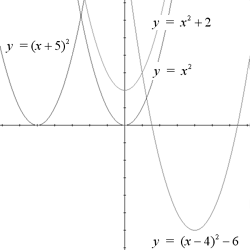The vertex is the maximum point for parabolas with a < 0 or minimum point for parabolas with a > 0. For parabolas of the form y = ax2, the vertex is (0,0). The vertex of a parabola can be shifted however, and this change is reflected in the standard equation for parabolas. Given a parabola y=ax2+bx+c, we can find the x-coordinate of the vertex of the parabola using the formula x=-b/2a. The standard equation has the form y = a(x - h)2 + k. The parabola y = ax2 is shifted h units to the right and k units upwards, resulting in a parabola with vertex (h,k).

Note: You may recognize this equation from the completing the square section above. The method of completing the square should be used to convert a parabola of general form to its standard form.

Note: For an example of graphing a quadratic equation, see question #2 in the Additional Examples section below.

1 | Solve the quadratic equation by completing the square
2 | Solve and graph the quadratic equation by completing the square

| Top of Page |

COURSE HOMEPAGES
MATH 1036
MATH 1037

FACULTY HOMEPAGES
Alex Karassev
Ted Chase